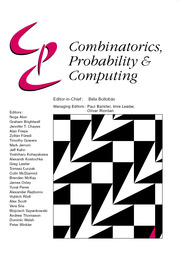Home
Hostname: page-component-7f7b94f6bd-mcrbk Total loading time: 0.265 Render date: 2022-06-29T02:54:56.144Z Has data issue: true Feature Flags: { "shouldUseShareProductTool": true, "shouldUseHypothesis": true, "isUnsiloEnabled": true, "useRatesEcommerce": false, "useNewApi": true } hasContentIssue trueCombinatorics, Probability and Computing

# On the size-Ramsey number of grid graphs

Published online by Cambridge University Press:  06 April 2021

*
*Corresponding author. Email: schacht@math.uni-hamburg.de

## Abstract

The size-Ramsey number of a graph F is the smallest number of edges in a graph G with the Ramsey property for F, that is, with the property that any 2-colouring of the edges of G contains a monochromatic copy of F. We prove that the size-Ramsey number of the grid graph on n × n vertices is bounded from above by n3+o(1).

## MSC classification

Type
Paper
Information
Combinatorics, Probability and Computing , September 2021 , pp. 670 - 685

## Access options

Get access to the full version of this content by using one of the access options below. (Log in options will check for institutional or personal access. Content may require purchase if you do not have access.)

## Footnotes

M. Miralaei was supported by the Ministry of Science, Research and Technology of Iran and part of the research was carried out during a visit to the University of Hamburg.

M. Schacht was partly supported by the European Research Council (PEPCo 724903).

## References

Allen, P., Böttcher, J., Hàn, H., Kohayakawa, Y. and Person, Y. Blow-up lemmas for sparse graphs. Submitted.Google Scholar
Alon, N. and Chung, F. R. K. (1988) Explicit construction of linear sized tolerant networks. Discrete Math. 72 1519.CrossRefGoogle Scholar
Beck, J. (1983) On size Ramsey number of paths, trees, and circuits, I. J. Graph Theory 7 115129.CrossRefGoogle Scholar
Beck, J. (1990) On size Ramsey number of paths, trees and circuits, II. In Mathematics of Ramsey Theory (Nešetřil, J. and Rödl, V., eds), pp. 3445. Springer.CrossRefGoogle Scholar
Bollobás, B. (2001) Random Graphs, Vol. 73 of Cambridge Studies in Advanced Mathematics, second edition. Cambridge University Press.CrossRefGoogle Scholar
Clemens, D., Jenssen, M., Kohayakawa, Y., Morrison, N., Mota, G. O., Reding, D. and Roberts, B. (2019) The size-Ramsey number of powers of paths. J. Graph Theory 91 290299.CrossRefGoogle Scholar
Conlon, D. and Nenadov, R. Size Ramsey numbers of triangle-free graphs with bounded degree. Preprint.Google Scholar
Dellamonica, D., Jr (2012) The size-Ramsey number of trees. Random Struct. Algorithms 40 4973.CrossRefGoogle Scholar
Dudek, A. and Prałat, P. (2015) An alternative proof of the linearity of the size-Ramsey number of paths. Combin. Probab. Comput. 24 551555.CrossRefGoogle Scholar
Dudek, A. and Prałat, P. (2017) On some multicolor Ramsey properties of random graphs. SIAM J. Discrete Math. 31 20792092.CrossRefGoogle Scholar
Erdős, P. (1981) On the combinatorial problems which I would most like to see solved. Combinatorica 1 2542.CrossRefGoogle Scholar
Erdős, P., Faudree, R. J., Rousseau, C. C. and Schelp, R. H. (1978) The size Ramsey number. Period. Math. Hungar. 9 145161.CrossRefGoogle Scholar
Friedman, J. and Pippenger, N. (1987) Expanding graphs contain all small trees. Combinatorica 7 7176.CrossRefGoogle Scholar
Gerke, S., Kohayakawa, Y., Rödl, V. and Steger, A. (2007) Small subsets inherit sparse ε-regularity. J. Combin. Theory Ser. B 97 3456.CrossRefGoogle Scholar
Haxell, P. E. and Kohayakawa, Y. (1995) The size-Ramsey number of trees. Israel J. Math. 89 261274.CrossRefGoogle Scholar
Haxell, P. E., Kohayakawa, Y. and Łuczak, T. (1995) The induced size-Ramsey number of cycles. Combin. Probab. Comput. 4 217239.CrossRefGoogle Scholar
Janson, S., Łuczak, T. and Ruciński, A. (2000) Random Graphs, Wiley-Interscience Series in Discrete Mathematics and Optimization. Wiley-Interscience.CrossRefGoogle Scholar
Javadi, R., Khoeini, F., Omidi, G. R. and Pokrovskiy, A. (2019) On the size-Ramsey number of cycles. Combin. Probab. Comput. 28 871880.CrossRefGoogle Scholar
Kohayakawa, Y. (1997) Szemerédi’s regularity lemma for sparse graphs. In Foundations of Computational Mathematics (Cucker, F. and Shub, M., eds), pp. 216230. Springer.CrossRefGoogle Scholar
Kohayakawa, Y. and Rödl, V. (2003) Regular pairs in sparse random graphs, I. Random Struct. Algorithms 22 359434.CrossRefGoogle Scholar
Kohayakawa, Y., Rödl, V., Schacht, M. and Szemerédi, E. (2011) Sparse partition universal graphs for graphs of bounded degree. Adv. Math. 226 50415065.CrossRefGoogle Scholar
Letzter, S. (2016) Path Ramsey number for random graphs. Combin. Probab. Comput. 25 612622.CrossRefGoogle Scholar
Rödl, V. and Duke, R. A. (1985) On graphs with small subgraphs of large chromatic number. Graphs Combin. 1 9196.CrossRefGoogle Scholar
Rödl, V. and Szemerédi, E. (2000) On size Ramsey numbers of graphs with bounded degree. Combinatorica 20 257262.Google Scholar
Szemerédi, E. (1978) Regular partitions of graphs. In Problèmes Combinatoires et Théorie des Graphes, Vol. 260 of Colloq. Internat. CNRS, pp. 399401. CNRS.Google Scholar

# Save article to Kindle

Note you can select to save to either the @free.kindle.com or @kindle.com variations. ‘@free.kindle.com’ emails are free but can only be saved to your device when it is connected to wi-fi. ‘@kindle.com’ emails can be delivered even when you are not connected to wi-fi, but note that service fees apply.

Find out more about the Kindle Personal Document Service.

On the size-Ramsey number of grid graphs
Available formats
×

# Save article to Dropbox

To save this article to your Dropbox account, please select one or more formats and confirm that you agree to abide by our usage policies. If this is the first time you used this feature, you will be asked to authorise Cambridge Core to connect with your Dropbox account. Find out more about saving content to Dropbox.

On the size-Ramsey number of grid graphs
Available formats
×

# Save article to Google Drive

To save this article to your Google Drive account, please select one or more formats and confirm that you agree to abide by our usage policies. If this is the first time you used this feature, you will be asked to authorise Cambridge Core to connect with your Google Drive account. Find out more about saving content to Google Drive.

On the size-Ramsey number of grid graphs
Available formats
×
×